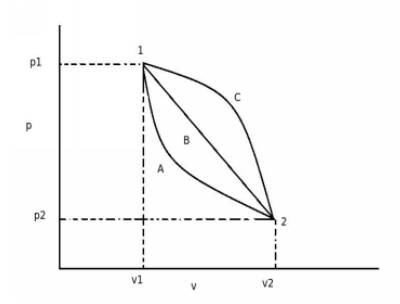# Change in Internal Energy of a System.

## A system changes its state from state 1 to state 2 through path A and returns from state 2 to state 1 through path B. Path C is also an alternate returning path from state 2 to state 1 as shown in figure. What will be the effect on change in internal energy of the system(ΔE), if the system undergoes these different paths A, B and C?a. ΔE of path C will be greater then ΔE of path B and path A
b. ΔE of path A and path C are equal but ΔE of path B is different than rest
c. ΔE of all the paths are equal
d. none of the above

Correct Answer : c. ΔE of all the paths are equal

Explanation :

According to the description of path given, through the path A and path B system undergoes cycle,
Writing the first law equation for path A,
QA = ΔEA + WA

and for path B,
QB = ΔEB + WB

The processes A and B together constitute a cycle, for which
(∑ W)cycle = (∑ Q)cycle
WA + WB = QA + QB
QA – WA = WB – QB
ΔEA = – ΔEB

Similarly, when the system returns from state 2 to state 1 through path C,
ΔEA = – ΔEC

Therefore,
ΔEB = ΔEC

Therefore above equations show that the change in internal energy between two states of the system is the same, no matter which path may system follow.